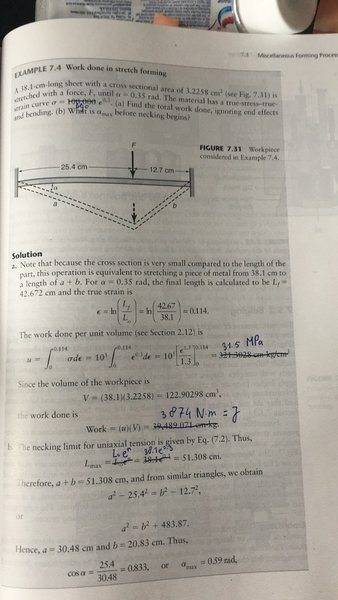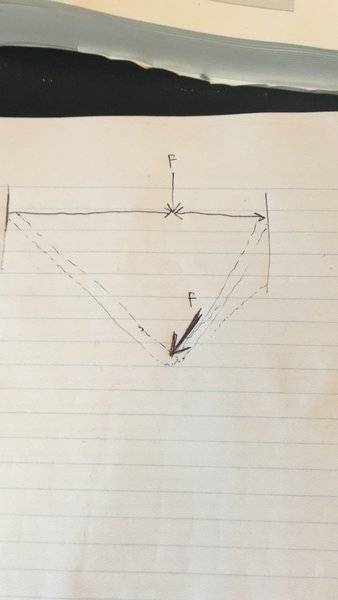# Deformation of a metal sheet with a tip force applied

## Homework Statement

Stretch forming[/B]
A 38.1 cm-long sheet with a cross sectional area of 3.2258 cm2 is stretched with a force, F, until alpha = 0.35 rad. The tip of the force is fixed to the strip by some means, thus maintaining the lateral position of the force. (The left portion of the strip will now be strained more than the right portion.) The material has a true stress-strain curve of sigma = 690 * epsilon 0.3
a) find the total work done, ignoring end effects and bending
b) What is alphamax before necking begins?

## Homework Equations

epsilon = ln(Lfinal/L0)

## The Attempt at a Solution

I can already calculate the work done by taking the integral to epsilon of the true stress-strain curve (which is the work done per unit volume) and thereafter multiplying this by the volume of the metal sheet. The problem is however that I do not know how long the sheet will be (a and b, see images attached) if the force would be attached to the tip and therefore maintaining the lateral position. In the image attached the tip of the force is not attached to the sheet and in the second image a quick drawing of the idea can be seen. It would be great if anyone could help me further!#### Attachments

Chestermiller
Mentor

NEW
It really is a point load! In the example, the load shifts a bit (for example due to lubrication or rollers), but in this case the tip of the force is attached to the sheet.

Chestermiller
Mentor
NEW
It really is a point load! In the example, the load shifts a bit (for example due to lubrication or rollers), but in this case the tip of the force is attached to the sheet.
How is the tip attached to the sheet?

How is the tip attached to the sheet?
Any information about how the tip is attached is lacking, it only states that the tip of the force is fixed to the strip 'by some means'

Chestermiller
Mentor
From their statement of the problem and their method of attack, it is pretty clear that they are describing knife-edge loading, not point loading.